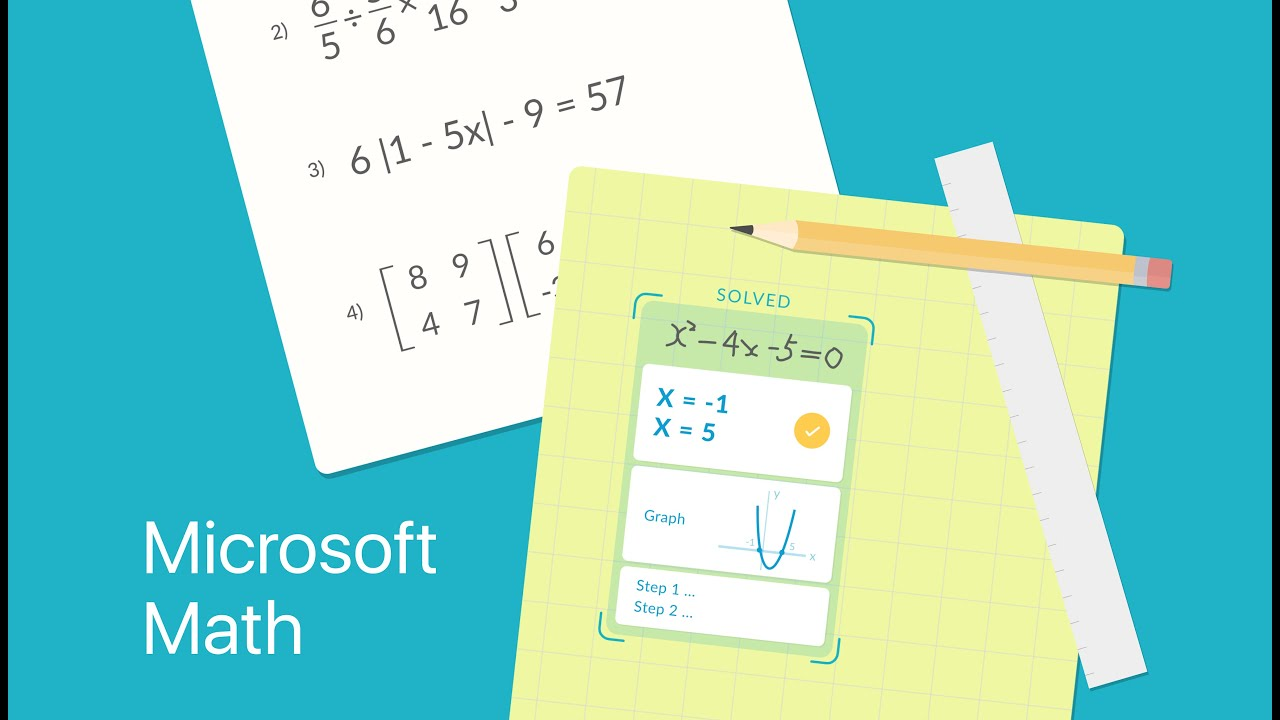Microsoft Math Solver is a math application from Microsoft that can solve maths and explains the resolution of answers. The app allows you to solve calculations from mobile phone photos, hand drawings, or by hand typing. Microsoft Math Solver is available for free for Android and iPhone (iOS) phones.

## My Opinion for Microsoft Math Solver Android Apps

Microsoft Math Solver Android apk has a quite simple user interface and easy to use: just point your camera phone at a handwritten or printed equation on paper and capture a photo. Then the application will identify the math problem and show its results immediately. The tool also displays a complete walkthrough of how the math was resolved, with detailed explanations of each topic.In addition to the camera, the user can write finger-on-screen equations. But I think this function may not be useful for people with large fingers and if their phones screen space is too small to write a complete math problem. However, recognition of drawn numbers is awesome, and the results are displayed immediately. That's what I like the most.

Another option is the specific keyboard for writing equations provided by the app. Here you can type numbers, letters, and math symbols to create more complex functions of math.

The app can solve problems of arithmetic, algebra, trigonometry, calculus, statistics, and other topics and also can answer questions involving complex numbers, factorization, root, matrices, determinants, average, median, among others.Explanations are displayed in several languages, including Portuguese. The super-easy thing is the user can accesses graphics and can search subject related videos to watch resolution tutorials within the app itself.

#### Pros of Microsoft Math Solver

• Resolve Maths Immediately
• Works in Multiple Languages
• Shows Step by Step Resolution

#### Cons of Microsoft Math Solver

• There is no (I think)

### Microsoft Math Solver can Solve

• Elementary math problems like arithmetic, real, complex numbers, LCM, GCD, factors, and roman numerals.
• Pre-Algebra related math problems like radicals and exponents, fractions, matrices, and determinants.
• Algebra related math problems like quadratic equations, the system of equations, inequalities, rational expressions, linear, quadratic and exponential graphs.
• Word problems on math concepts, number theory, probability, volume, surface area. Basic Calculus: Summations, Limits, derivatives, and integrals.
• Lastly, Statistics related math problems like Mean, Median, Mode, Standard Deviation, permutations, and combinations.

Details of Microsoft Math Solver
Developer Microsoft Corporation
Minimum OSAndroid 5.0 and above
Size22.85
Installs100,000+
Released onDec 6, 2019
Current Version1.0.30